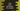# Java Program to count the divisors of a number## Write a Java program to count the number of divisors of a number :

In this tutorial, I will show you how to find the total number of divisors for a number starting from 1 using Java. Let’s move through the steps first :

1. We are using one different method ’findCountOfDivisors(int no)’ to find the count. This method takes one number as the parameter and finds the divisor count for that number.
2. First of all, take the number from the user by using the ’Scanner’ class.
3. Now, pass the number to the ’findCountOfDivisors’ method.
4. Create one variable ’result’ with the initial value as ’0’. This variable will store the final count.
5. Start one ’for’ loop . This loop will start from i=1 and run till ’i’ becomes equal to the number. ’i’ will increase each time by 1.
6. Check if ’i’ can divide the number or not. If ’true’, increase the ’result’ variable. That means, since ’i’ is starting from 1 to that number, we will check for each number from ’1 to that number’ if it can divide the number or not.
7. After the loop is completed, print the ’result

### Java Program :

``````/*
* Copyright (C) 2021 codevscolor
*
* Licensed under the Apache License, Version 2.0 (the "License");
* you may not use this file except in compliance with the License.
* You may obtain a copy of the License at
*
*
* Unless required by applicable law or agreed to in writing, software
* distributed under the License is distributed on an "AS IS" BASIS,
* WITHOUT WARRANTIES OR CONDITIONS OF ANY KIND, either express or implied.
* See the License for the specific language governing permissions and
* limitations under the License.
*/

import java.util.Scanner;

/**
* Example Class
*/
public class ExampleClass {

/**
* System.out.println utility method
*
* @param value : value to print
*/
static void print(String value) {
System.out.println(value);
}

/**
* Method to find the count of divisors of a number
*
* @param no : number to find the count of divisors
* @return : no return value, just print the result
*/
static void findCountOfDivisors(int no) {

//variable to store the result
int result = 0;

//start a loop and check for each number if it can divide the given number
for (int i = 1; i <= no; i++) {
if (no % i == 0) {
//if the reminder is zero, increment the result variable
result++;
}
}

print("Total number of divisors : " + result);

}

/**
* main method for this class
*/
public static void main(String[] args) {

//variable to get the user input
int no;

//scanner object to get the user input
Scanner scanner = new Scanner(System.in);

print("Enter a number : ");

//read the user-input number
no = scanner.nextInt();

findCountOfDivisors(no);

}
}``````

### Sample Output :

``````Enter a number :
365
Total number of divisors : 4``````